### Subhash R. Lele. 2006. Sampling variability and estimates of density dependence: a composite-likelihood approach. Ecology 87:189–202.

Appendix B. Mathematical details of the computation of the means, variances, and covariance of the nonstationary Gompertz process.

In the following I give full details of the calculations of the means, variances, and covariance of the nonstationary Gompertz process.

Let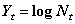and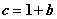. With this notation, we can rewrite the Nonstationary Gompertz model as follows: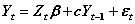. Hence we can write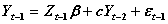. Substituting this expression in the first expression, we can rewrite the first expression as: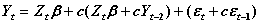. Simplifying and repeating similar substitutions, we obtain: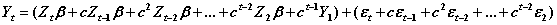.

Using this and the fact that environmental noise has mean 0, variance etc., it follows that: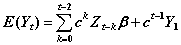,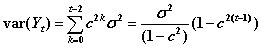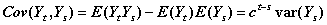where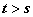.

Last expression is simple algebraic simplification by noting the following results: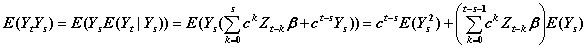and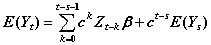.

[Back to E087-008]